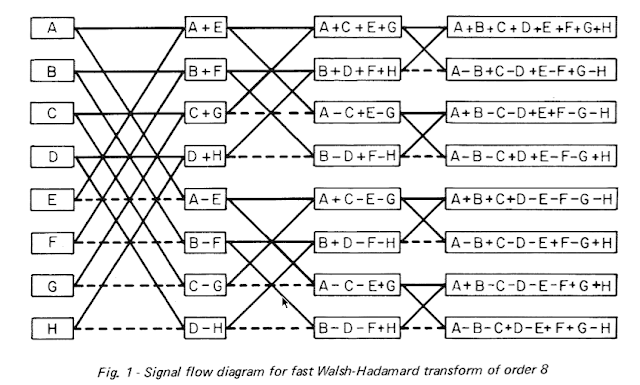The 2-point WHT of [a,b] is [a+b,a-b]. The operation is self-inverse

after accounting for a constant multiplier [(a+b)+(a-b),(a+b)-(a-b)] = [2a,2b] .

Scaling each (+-) operation by 1/sqr(2) leaves the vector length unchanged.

The 4-point algorithm of [a,b,c,d] can be done by first doing

[a+b,a-b] and [c+d,c-d] and then forming the sum and difference of the sum

terms and the sum and difference of the difference terms.Next

Random Projection AI
S6Regen Code
Similarity Alignment